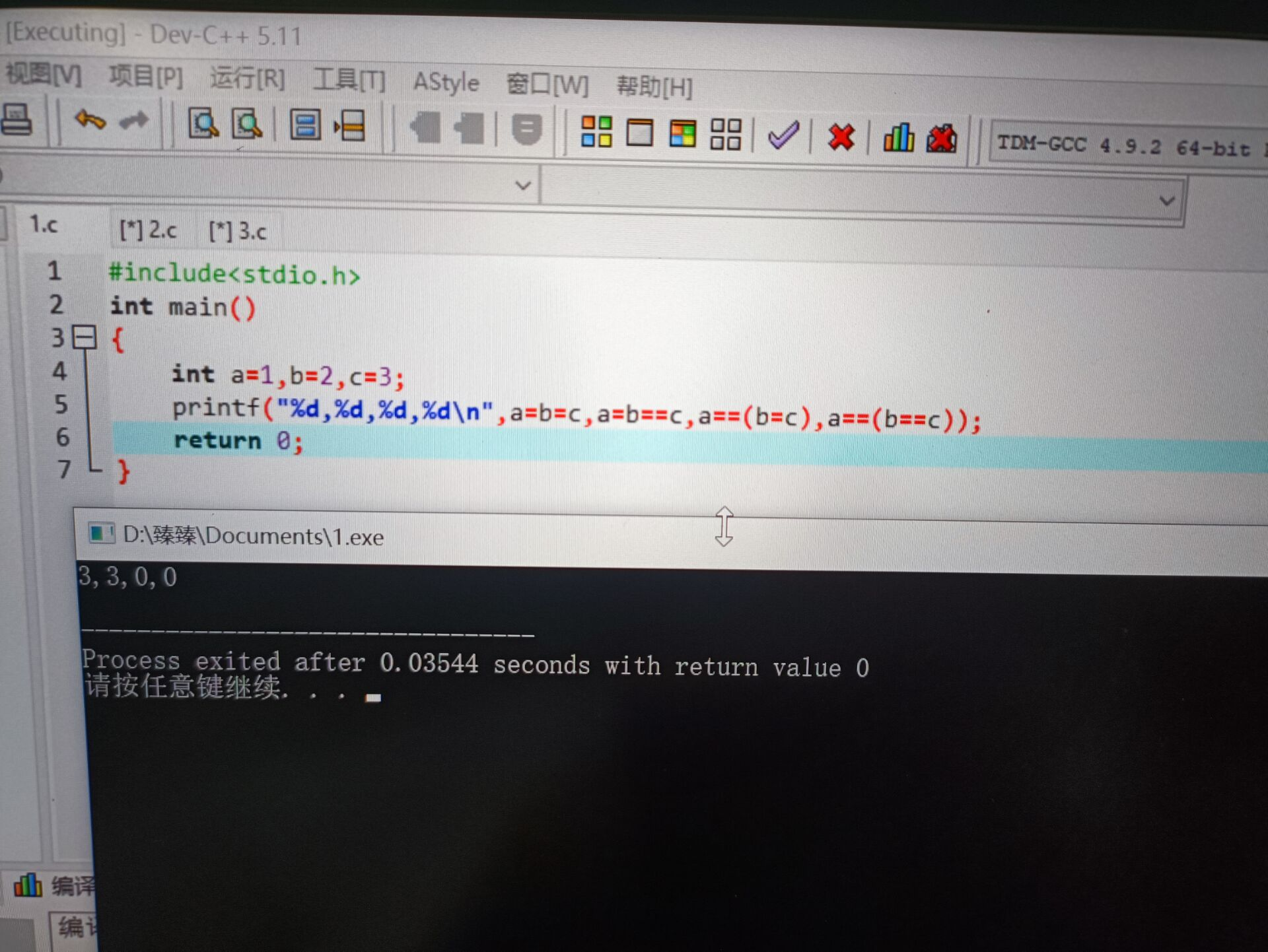2021-11-09 22:15

关于Dev-C++5.11运行以下逻辑判断代码结果错误，求详细解答

#include<stdio.h>
int main()
{
int a=1,b=2,c=3;
printf("%d,%d,%d,%d\n",a=b=c,a=b==c,a==(b=c),a==(b==c));
return 0;
}• 好问题 提建议
• 收藏